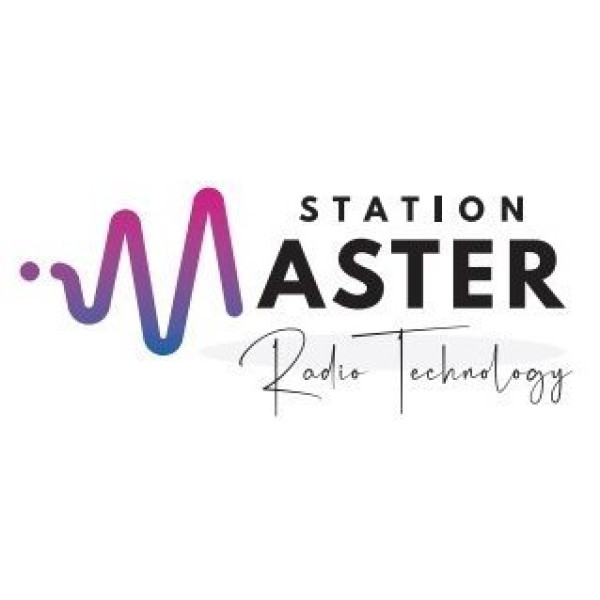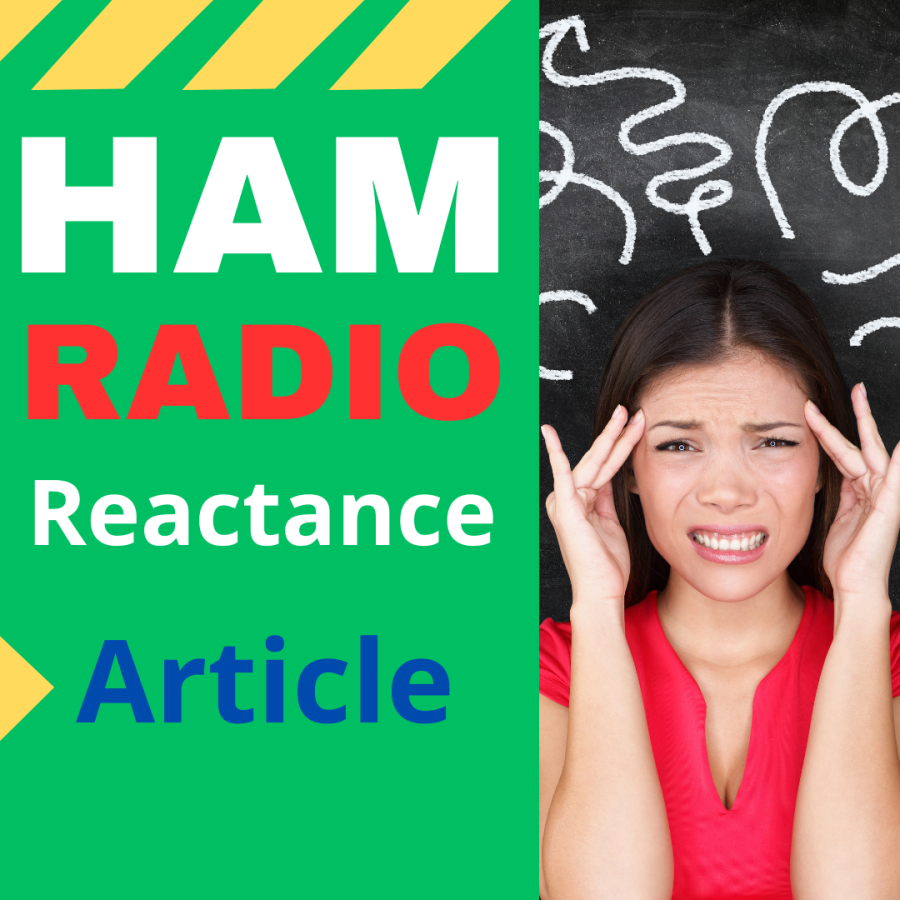Stuart E. Green's Article 6 Months Ago - last edit 6 Months Ago

# Antenna Reactance Explained

Antenna reactance is an important concept in the world of ham radio. Understanding antenna reactance is critical for optimizing the performance of your antenna and improving the quality of your transmissions. In this article, we'll explain what antenna reactance is, how it affects antenna performance, and how to calculate and adjust antenna reactance.

Reactance is a measure of the opposition of an electrical circuit to a change in current or voltage. In the context of an antenna, reactance is the amount of opposition that the antenna presents to the flow of current. Antenna reactance is a combination of two types of reactance: capacitive reactance and inductive reactance.

Capacitive reactance occurs when the antenna behaves like a capacitor, storing electrical charge between two conductors separated by a dielectric material. Inductive reactance occurs when the antenna behaves like an inductor, storing electrical energy in a magnetic field that surrounds a conductor.

The reactance of an antenna can affect the efficiency of the antenna, the quality of the transmitted signal, and the range of the radio station. When an antenna has a high level of reactance, it can cause energy to be reflected back from the antenna, reducing the amount of energy that is radiated into free space.

To calculate the reactance of an antenna, you will need to know the frequency of operation and the electrical properties of the antenna. The formula for calculating reactance is:

X = 2πfL - 1/(2πfC)

Where X is the reactance in ohms, f is the frequency in hertz, L is the inductance in henries, and C is the capacitance in farads.

If the reactance of an antenna is too high, it can be adjusted by adding or removing capacitance or inductance from the antenna. This can be done by adding or removing components such as capacitors, inductors, or coils from the antenna circuit. By adjusting the reactance of the antenna, you can improve the efficiency of the antenna and increase the amount of energy that is radiated into free space.{{comment.user.my_callsign.toUpperCase()}} {{comment.message}}